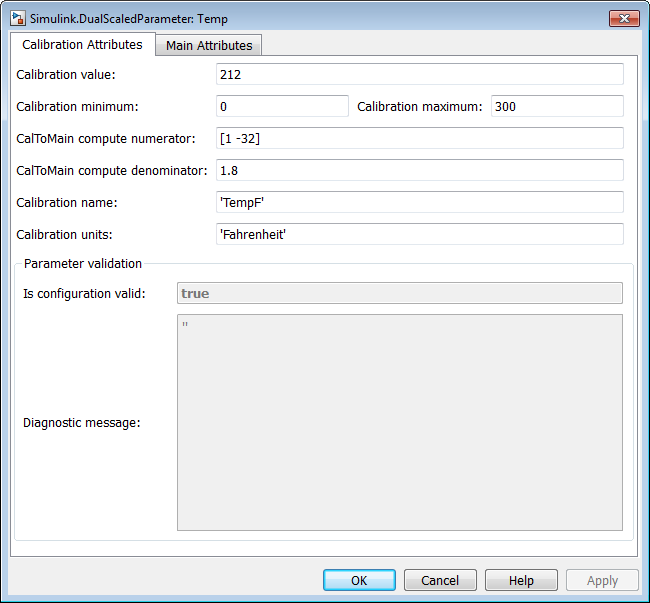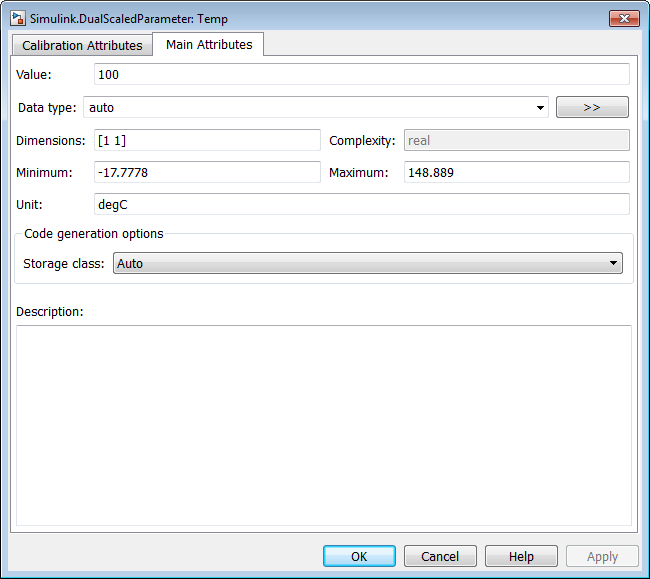Specify name, value, units, and other properties of Simulink dual-scaled parameter

## Description

Use `Simulink.DualScaledParameter` so that you can define an object that stores two scaled values of the same physical value.

For example, for temperature measurement, you can store a Fahrenheit scale and a Celsius scale with conversion defined by a computation method that you provide. Given one scaled value, the `Simulink.DualScaledParameter` computes the other scaled value using the computation method.

A dual-scaled parameter inherits some properties from the `Simulink.Parameter` class. A dual-scaled parameter has:

• A calibration value. The value that you prefer to use.

• A main value. The real-world value that Simulink® uses.

• An internal stored integer value. The value that is used in the embedded code.

You can use `Simulink.DualScaledParameter` objects in your model for both simulation and code generation. The parameter computes the internal value before code generation via the computation method. This offline computation results in leaner generated code.

If you provide the calibration value, the parameter computes the main value using the computation method. This method can be a first-order rational function.

`$y=\frac{ax+b}{cx+d}$`

• `x` is the calibration value.

• `y` is the main value.

• `a` and `b` are the coefficients of the CalToMain compute numerator.

• `c` and `d` are the coefficients of the CalToMain compute denominator.

If you provide the calibration minimum and maximum values, the parameter computes minimum and maximum values of the main value. Simulink performs range checking of parameter values. The software alerts you when the parameter object value lies outside a range that corresponds to its specified minimum and maximum values and data type.

## Creation

Create a `Simulink.DualScaledParameter` object:

• By using the Model Data Editor. Instead of creating a `Simulink.Parameter` object, create a `Simulink.DualScaledParameter` object.

• By using the Model Explorer:

1. In the Model Hierarchy pane, select a workspace or data dictionary.

3. In the Model Explorer — Select Object dialog box, set Object class to `Simulink.DualScaledParameter`.

• By using the `Simulink.DualScaledParameter` function, described below.

### Syntax

``DSParam = Simulink.DualScaledParameter``

### Description

example

````DSParam = Simulink.DualScaledParameter` returns a `Simulink.DualScaledParameter` object with default property values.```

## Properties

expand all

For information about properties in the property dialog box of a `Simulink.DualScaledParameter` object, see Simulink.DualScaledParameter Property Dialog Box.

Calibration value of this parameter, specified as a finite, real, double number. This value represents the value that you prefer to use.

Before specifying `CalibrationValue`, you must specify `CalToMainCompuNumerator` and `CalToMainCompuDenominator` to define the computation method. The parameter uses the computation method and the calibration value to calculate the main value that Simulink uses.

Corresponds to Calibration value in the property dialog box.

Example: `5.34`

Data Types: `double`

Calibration minimum value of this parameter, specified as a finite, real, double, scalar number. The default value, `[]`, means the minimum is unspecified.

Before specifying `CalibrationMin`, you must specify `CalToMainCompuNumerator` and `CalToMainCompuDenominator` to define the computation method. The parameter uses the computation method and the calibration minimum value to calculate the minimum or maximum value that Simulink uses. A first order rational function is strictly monotonic, either increasing or decreasing. If it is increasing, setting the calibration minimum sets the main minimum value. If it is decreasing, setting the calibration minimum sets the main maximum.

If the parameter value is less than the minimum value or if the minimum value is outside the range of the parameter data type, Simulink generates a warning. In these cases, when updating the diagram or starting a simulation, Simulink generates an error.

Corresponds to Calibration minimum in the property dialog box.

Example: `10.51`

Data Types: `double`

Calibration maximum value of this parameter, specified as a finite, real, double, scalar number. The default value, `[]`, means the maximum is unspecified.

Before specifying `CalibrationMax`, you must specify `CalToMainCompuNumerator` and `CalToMainCompuDenominator` to define the computation method. The parameter uses the computation method and the calibration maximum value to calculate the corresponding maximum or minimum value that Simulink uses. A first order rational function is strictly monotonic, either increasing or decreasing. If it is increasing, setting the calibration maximum sets the main maximum value. If it is decreasing, setting the calibration maximum sets the main minimum.

If the parameter value is less than the minimum value or if the minimum value is outside the range of the parameter data type, Simulink generates a warning. In these cases, when updating the diagram or starting a simulation, Simulink generates an error.

Corresponds to Calibration maximum in the property dialog box.

Example: `-10.51`

Data Types: `double`

Numerator coefficients of the computation method, specified as a scalar number or vector of values for the numerator coefficients `a` and `b` of the first-order linear equation:

`$y=\frac{ax+b}{cx+d}$`

The default value is `[]` (unspecified). Specify finite, real, double scalar values for `a` and `b`. For example, `[1 1]` or, for reciprocal scaling, `1`.

Once you have applied `CalToMainCompuNumerator`, you cannot change it.

Corresponds to CalToMain compute numerator in the property dialog box.

Example: `[1 1]`

Example: `1`

Data Types: `double`

Denominator coefficients of the computation method, specified as a scalar number or vector of values for the denominator coefficients `c` and `d` of the first-order linear equation:

`$y=\frac{ax+b}{cx+d}$`

The default value is `[]` (unspecified). Specify finite, real, double scalar values for `c` and `d`. For example, `[1 1]`.

Once you have applied `CalToMainCompuDenominator`, you cannot change it.

Corresponds to CalToMain compute denominator in the property dialog box.

Example: `[1 1]`

Data Types: `double`

Name of the calibration parameter, specified as a character vector.

Corresponds to Calibration name in the property dialog box.

Example: `'This is a calibration parameter.'`

Data Types: `char`

Measurement units for this calibration parameter's value, specified as a character vector.

Corresponds to Calibration units in the property dialog box.

Example: `'Fahrenheit'`

Data Types: `char`

Information about the validity of the object configuration, returned as `true` (valid) or `false` (invalid). If Simulink detects an issue with the configuration, it sets this field to `false` and provides information in the `DiagnosticMessage` property.

Corresponds to Is configuration valid in the property dialog box.

Data Types: `logical`

Diagnostic information about an invalid object configuration, returned as a character vector. If you specify invalid property settings, Simulink displays a message in this field. Use the diagnostic information to help you fix an invalid configuration issue.

Corresponds to Diagnostic message in the property dialog box.

Data Types: `char`

## Examples

collapse all

Create a `Simulink.DualScaledParameter` object that stores a temperature as both Fahrenheit and Celsius.

Create a `Simulink.DualScaledParameter` object.

```Temp = Simulink.DualScaledParameter; ```

Set the computation method that converts between Fahrenheit and Celsius.

```Temp.CalToMainCompuNumerator = [1 -32]; Temp.CalToMainCompuDenominator = [1.8]; ```

Set the value of the temperature that you want to see in Fahrenheit.

`Temp.CalibrationValue = 212`
```Temp = DualScaledParameter with properties: CalibrationValue: 212 CalibrationMin: [] CalibrationMax: [] CalToMainCompuNumerator: [1 -32] CalToMainCompuDenominator: 1.8000 CalibrationName: '' CalibrationDocUnits: '' IsConfigurationValid: 1 DiagnosticMessage: '' Value: 100 CoderInfo: [1x1 Simulink.CoderInfo] Description: '' DataType: 'auto' Min: [] Max: [] Unit: '' Complexity: 'real' Dimensions: [1 1]```

The `Simulink.DualScaledParameter` calculates `Temp.Value` which is the value that Simulink uses. `Temp.CalibrationValue` is `212` (degrees Fahrenheit), so `Temp.Value` is `100` (degrees Celsius).

Name the value and specify the units.

```Temp.CalibrationName = 'TempF'; Temp.CalibrationDocUnits = 'Fahrenheit';```

Set calibration minimum and maximum values.

```Temp.CalibrationMin = 0; Temp.CalibrationMax = 300; ```

If you specify a calibration value outside this allowable range, Simulink generates a warning.

Specify the units that Simulink uses.

`Temp.Unit = 'degC';`

`open Temp`The Calibration Attributes tab displays the calibration value and the computation method that you specified.

In the dialog box, click the Main Attributes tab.## Version History

Introduced in R2013b